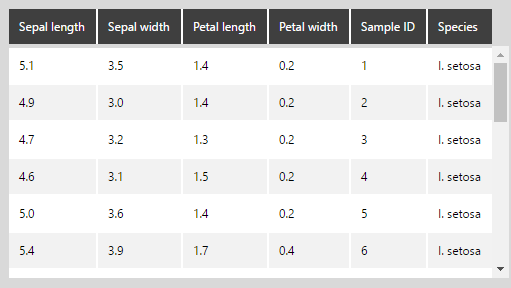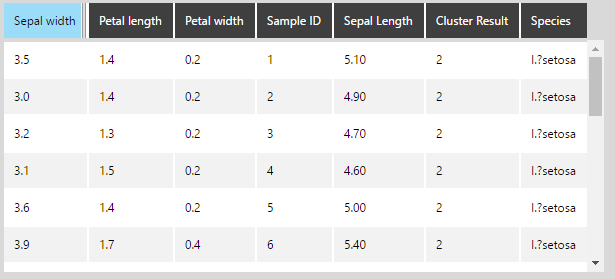# Clustering predictions using R language analysis

Contents[Hide]

## 1. Overview

This article shows an example of using R for analysis, creating clusters using a K-means model. K-means clustering aims to partition a number (n) of observations into a number (k) of clusters, in which each observation belongs to the cluster with the nearest mean, serving as a prototype of the cluster. Clustering can help identify different groups in your data that should receive special treatment based on their characteristics (for example, a defined custom marketing campaign for a certain cluster).

Important
This article is intended as an example of how to use R Language Analysis and run a custom formula. For the general K-Means Clustering formula, we recommend using the one existing in Dundas BI.

## 2. Prepare the data

The following example uses the Iris Flower Data Set. This data set contains the sepal and petal dimensions, which are used to predict the species of the Iris plant by classifying the data points into different clusters.

If necessary, first install and configure R.

If you are using SQL Server 2016, you can install the R add-on called the Advanced Analytics extension on the SQL server. If you choose to use this option, you do not need to install the R server and configure it for Dundas BI.

## 3. Create the data cube using R server

Create a data cube that retrieves the data. You can choose to have the input based on Excel, SQL, or any other source.

For example, the following data has been retrieved from the data set using a Manual Select transform.Iris Flower Data in the data cube

From the Insert Other option in the toolbar, add an R Language Analysis transform after the Select transform.

Configure the R Language Analysis transform and add the following script:

```#Define an argument for each column in the data set.
arg1 <- \$PetalLength\$
arg2 <- \$PetalWidth\$
arg3 <- \$SepalLength\$
arg4 <- \$SepalWidth\$
arg5 <- \$Species\$
arg6 <- \$SampleID\$

#Use the K-means algorithm to classify the Petal Length, Petal Width, Sepal Length, and Sepal Width into three clusters
result <- kmeans(data.frame(arg1, arg2, arg3, arg4), 3);

#Dundas BI requires that the output be in the form of a data frame
output <- data.frame(arg1, arg2, arg3, arg4, result\$cluster, arg5, arg6);
```

This script is using the K-means clustering algorithm, which requires multiple arguments and defines the number of clusters to generate out of those variables.

Note
The number of clusters is an input parameter (in this example we used 3 as the number of clusters). An inappropriate choice of k may yield poor results. That is why, when using the K-means model, it is important to run diagnostic checks to determine the optimal number of clusters in the data set.

In the same configuration panel, click Define Element Placeholders and add the placeholders for all the arguments defined in the script. Make sure the column names match with something that the R script recognizes.

Open the data preview. You can see that the new result.cluster column, which is classifying each data point into clusters 1, 2, or 3.

Note
arg5, the specie we are trying to predict, isn't necessary for this example but is used here to help us assess the accuracy of the cluster detection by comparing it to known clusters - in this case the species of the Iris flower.

## 4. Create the data cube using SQL Server 2016

If you are using SQL Server 2016 and have the Advanced Analytics extension installed, you can use the R script as part of the SQL query in the Manual Select node.

Create a Manual Select data cube using the following script:

```exec sp_execute_external_script
@language = N'R'
, @script = N'
df <- data.frame(InputDataSet)
arg1 <- df\$SepalLength
arg2 <- df\$SepalWidth
arg3 <- df\$PetalLength
arg4 <- df\$PetalWidth
arg5 <- df\$Species
arg6 <- df\$SampleID

result <- kmeans(data.frame(arg1, arg2, arg3, arg4), 3);
OutputDataSet <- data.frame(arg1, arg2, arg3, arg4, result\$cluster, arg5, arg6);
'
, @input_data_1 = N'
Select 5.1 As [SepalLength], 3.5 As [SepalWidth], 1.4 As [PetalLength], 0.2 As [PetalWidth], ''I. setosa'' as [Species], 1 As [SampleID] UNION ALL
Select 4.9, 3, 1.4, 0.2, ''I. setosa'', 2 UNION ALL
Select 4.7, 3.2, 1.3, 0.2, ''I. setosa'', 3 UNION ALL
…………
Select 6.5, 3, 5.2, 2, ''I. virginica'', 148 UNION ALL
Select 6.2, 3.4, 5.4, 2.3, ''I. virginica'', 149 UNION ALL
Select 5.9, 3, 5.1, 1.8, ''I. virginica'', 150'
WITH RESULT SETS (([Sepal Length] float, [Sepal width] float, [Petal length] float, [Petal width] float, [Cluster Result] int, [Species] varchar(MAX),[Sample ID] int));
```

This script specifies the language (i.e., R) and executes the K-means algorithm as a standard stored procedure. It also includes the Select statements for retrieving the data points from the database.

The same result is obtained as with using an R server:Cluster result is the same as using R server

## 5. Use the results

From the data cube created above, select the two measures, and add Sample ID to Rows in the Data Analysis Panel of a metric set.

Add the Predicted Cluster dimension to Columns, so that each Sample ID is grouped by the cluster.

Revisualize to a Point Chart. You will see that all the samples are grouped by cluster.

Note
The clusters may change every time the data is retrieved (assuming that data is not cached). The reason for this is that the K-means algorithm isn't a deterministic algorithm, as it has a random component used to define the clusters.